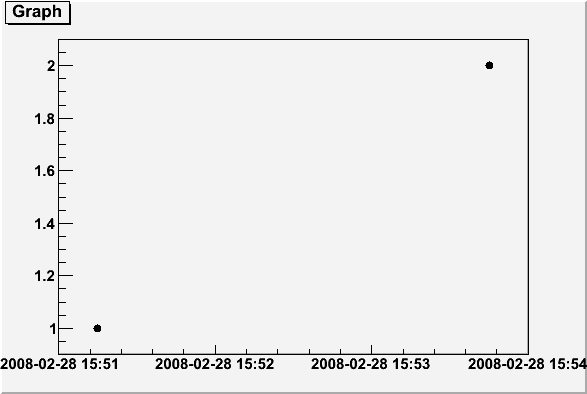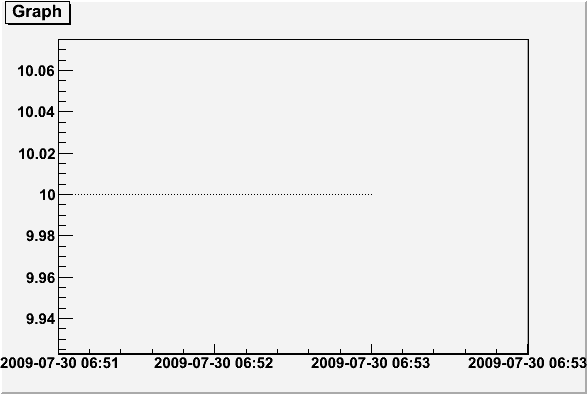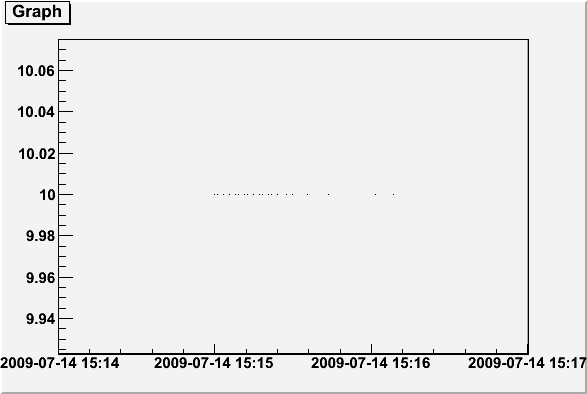# Data not correct

I have some problems to display date and time on a TGraph: my points are not correctly positioned on the time scale.

I tried this simple example: root.cern.ch/drupal/content/how- … time-units (the last one).

I attach the TGraph plot, as you can see the first point is not on 15:52:00 but on 15:51:00, the second is not on 15:53:00I’ll check…

The macro on the “how-to” page had mistakes.

1. the x and y vectors should be double not float
2. a “->” was used instead of a “.” on the last line.

But the real mistake was 1) … I fixed it on the “how-to” page. Thanks for reporting.

I tried with more data (look at data_root.txt attached).

I used a python script

``````import ROOT
import re
from array import array

f = open("data_root.txt")
r = re.compile(r"[A-Za-z]{3} (\d+) (\d{2}):(\d{2}):(\d{2})")
time = array('d',[])
y = array('d',[])
for line in f:
m = r.match(line)
d,h,m,s = map(int,m.groups())
time.append(ROOT.TDatime(2009,7,d,h,m,s).Convert())
y.append(10.)

gr=ROOT.TGraph(len(time), time, y)

gr.Draw("AP")
gr.GetXaxis().SetTimeDisplay(1)
gr.GetXaxis().SetNdivisions(-503)
gr.GetXaxis().SetTimeFormat("%Y-%m-%d %H:%M")
gr.GetXaxis().SetTimeOffset(0,"gmt")

raw_input()``````

the first data must be on Jul 14 16:15:50, the last one on Jul 30 07:53:01
but looking at the attached plot there are some problems. The time is shifted by 1 hour!

I tried also with a c++ code:

``````#include <fstream>
#include <iostream>
#include <string>
#include <vector>

#include "TDatime.h"
#include "TGraph.h"
#include "TApplication.h"
#include "TCanvas.h"
#include "TAxis.h"

int main(int argc, char **argv){
TApplication app("app", &argc, argv);
std::ifstream f("data_root.txt");
std::string line;
std::string month;
std::vector<double> time;
std::vector<double> y;
int day, hour, min, second;
while(!f.eof())
{
char c;
f >> month >> day >> hour >> c >> min >> c >> second ;
time.push_back(TDatime::TDatime(2009,7,day,hour,min,second).Convert());
y.push_back(10.);
}

TGraph gr(time.size(), &(time), &(y));
TCanvas canvas("canvas");
gr.Draw("AP");
gr.GetXaxis()->SetTimeDisplay(1);
gr.GetXaxis()->SetNdivisions(-503);
gr.GetXaxis()->SetTimeFormat("%Y-%m-%d %H:%M");
gr.GetXaxis()->SetTimeOffset(0,"gmt");

app.Run();
}``````

but the result is exaclty the same.data_root.txt (161 KB)

is the example in the “how to” ok for you after the mods I suggested you ?

yes, it is